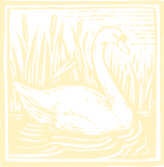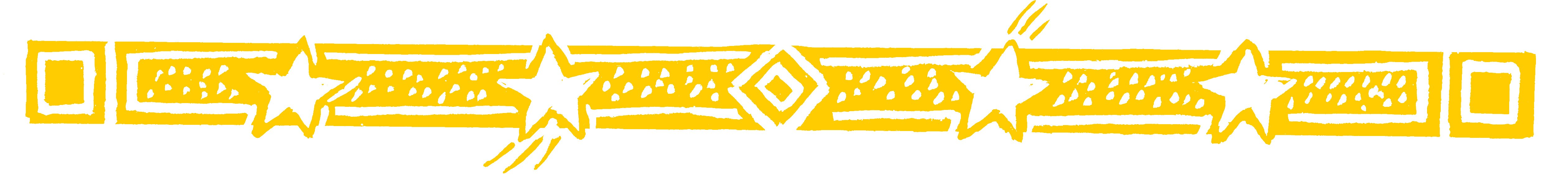Show your child this truly beautiful method of dividing by 9.

• 23 ÷ 9 = 2 remainder 5

The first figure of 23 is 2, and this is the answer.
The remainder is just 2 and 3 added up!

• 43 ÷ 9 = 4 remainder 7

The first figure 4 is the answer
and 4 + 3 = 7 is the remainder - could it be easier?

Question Comment !
 Tutorial Title Question Title No Questions 0 Questions Asked 0 Skipped Questions 0 Correctly Answered 0 Wrongly Answered 0 Total Question Attempts 0 Current Question Attempts 0

Skip Question

Reset Test

Halt Test

 No Questions 0 Questions Asked 0 Skipped Questions 0 Correctly Answered 0 Wrongly Answered 0 Total Question Attempts 0

Time Taken

CloseDelight Your Child (1)

• 134 ÷ 9 = 14 remainder 8

The answer consists of 1,4 and 8.
1 is just the first figure of 134.
4 is the total of the first two figures 1+ 3 = 4,
and 8 is the total of all three figures 1+ 3 + 4 = 8.Delight Your Child (2)…otherwise than
according to her
[mathematics]
order nothing can
exist, and nothing
takes place in